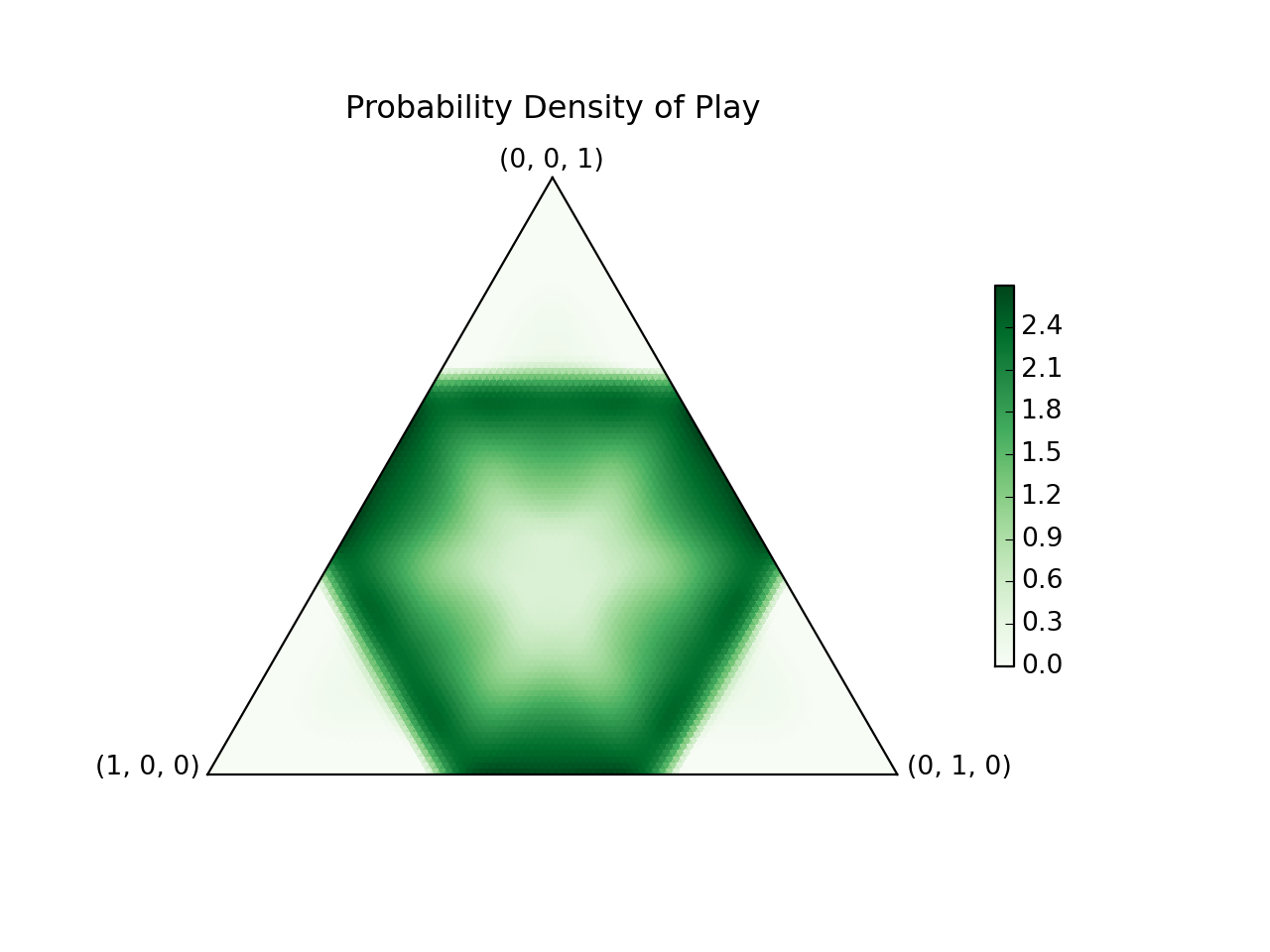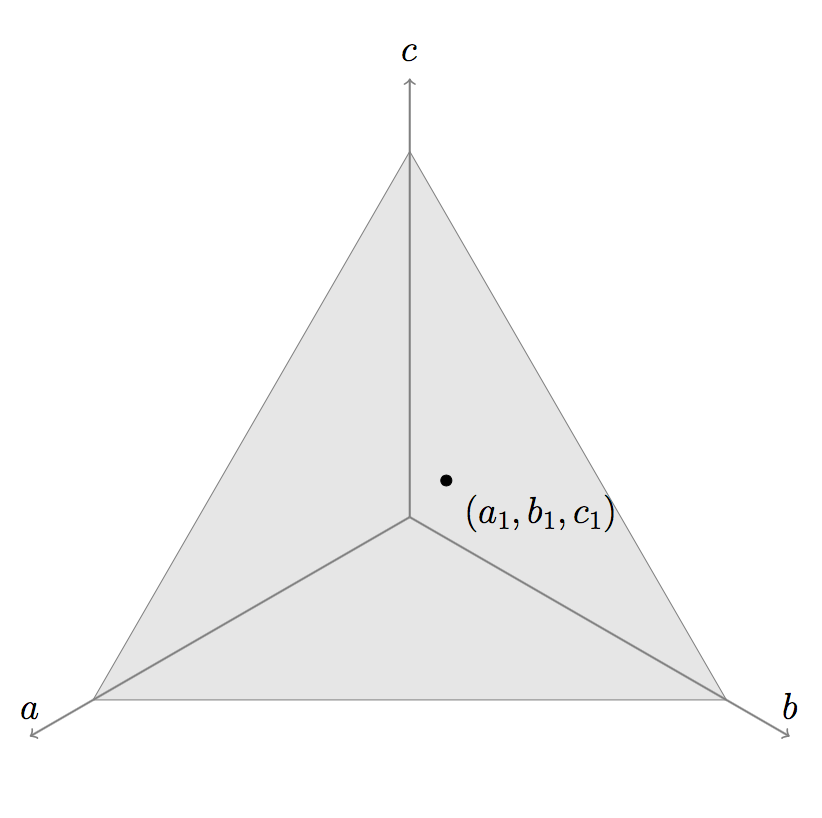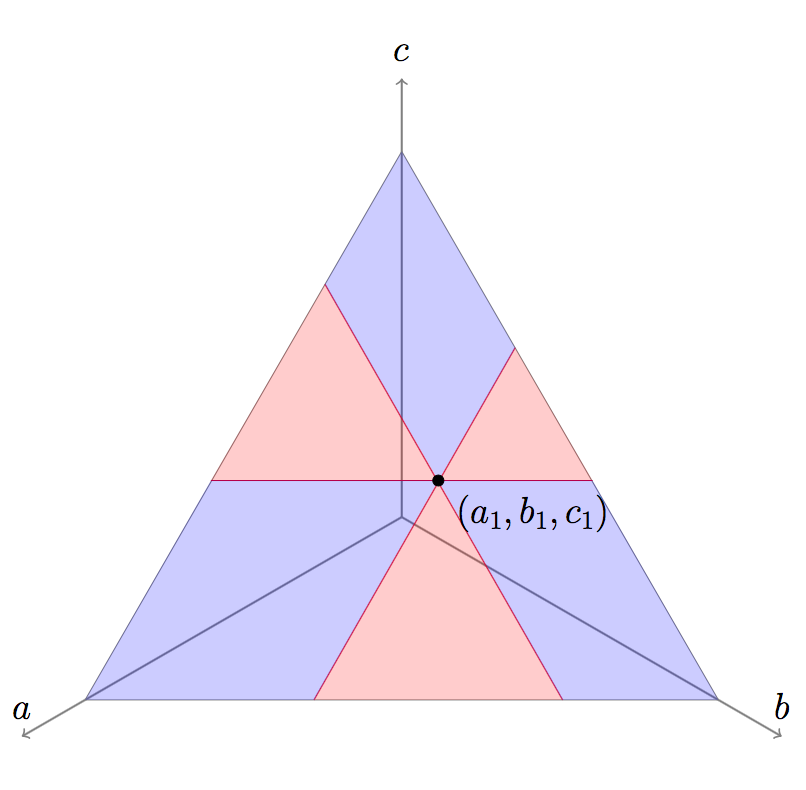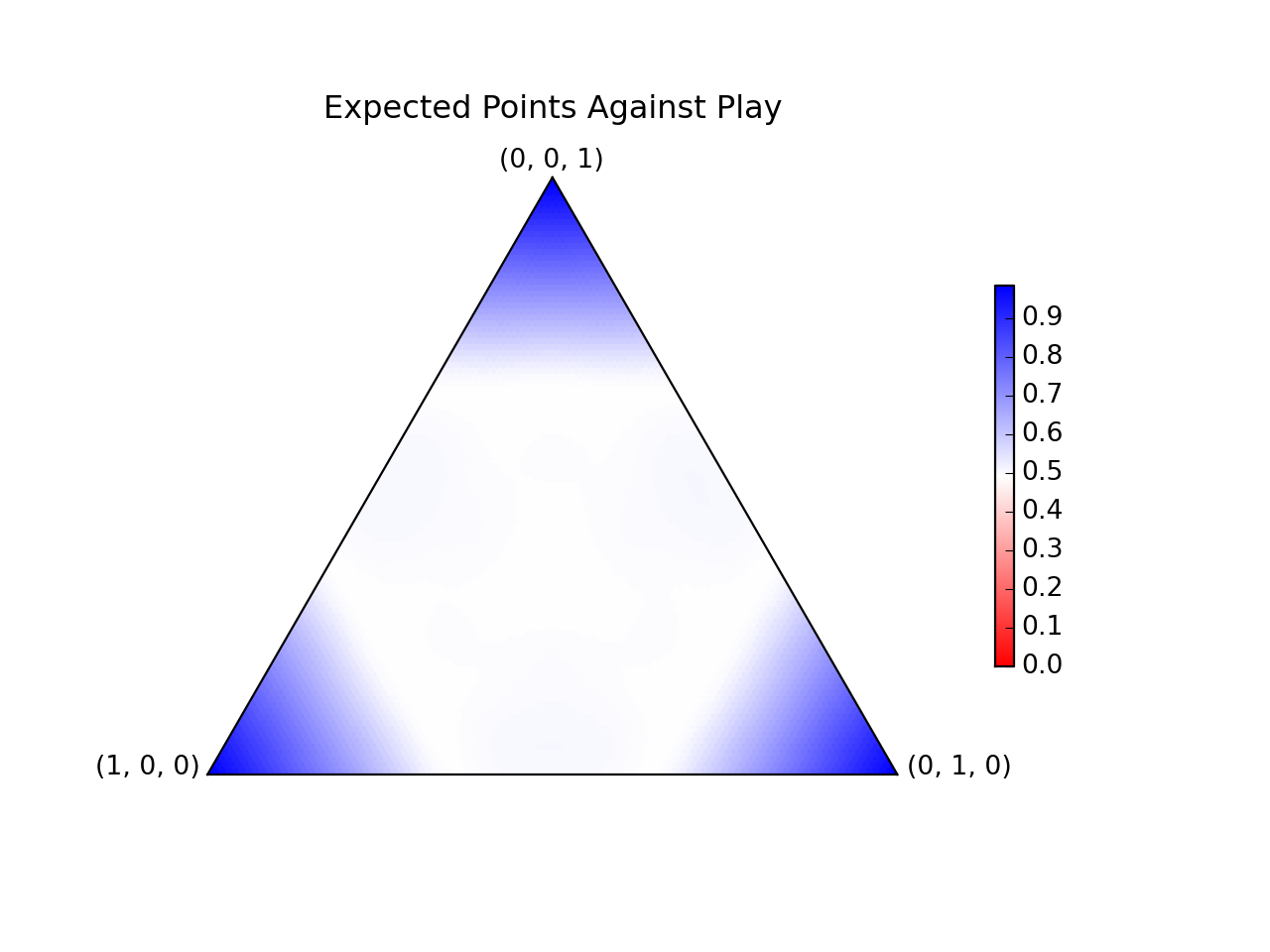Graph all the things

analyzing all the things you forgot to wonder about

# Humans versus Zombies

2015-10-09

A friend and former college Humans vs. Zombies (HvZ) moderator gave me this problem: suppose we set up a game where an equal number of humans and zombies can disperse among 3 battlefields, and whichever faction wins on at least 2 battlefields is the overall winner. To simplify things, each team has the same number of total players, and each battle is won by the team with more players on that battlefield. What is the optimal way to allocate your players?

Every allocation is beaten by some other allocation. For instance, if I send my people equally to all battles, my opponent can win with. That strategy in turn could be beaten by, which is beaten by.

I solved the game numerically, and here's what the solution looks like:It's pretty cool, but let's first learn rock, paper, scissors.

### Rock, Paper, Scissors (RPS)

In RPS, rock beats scissors, scissors beats paper, and paper beats rock. You get one point for beating the other person's choice, 1/2 a point for choosing the same thing, and 0 points for getting beaten.

I have a friend Mimee (who also helped me think through part of this problem) whose RPS strategy is to always play rock. This is great against anyone predisposed to play scissors, but doesn't work very well after people catch on. You can beat this strategy 100% of the time by playing paper.

My favorite strategy is to play whatever the other person's previous play would have beat. This works against many people because they rarely play the same thing twice in a row, but it still isn't a very good strategy. It would loose about 100% of the time to a stubborn person who keeps playing rock.

For competitive play, the best technique is of course to choose randomly, with equal probability to rock, paper, and scissors. This way, no matter what technique the opponent uses, they can't get more points than you on average. In general, if your chances of playing rock, paper, or scissors arerespectively, you have an expected outcome ofagainst someone playing rock, and so forth. This can be simply written aswhereis your expected number of points against rock, and so forth. We can derive the optimal technique by demanding that we average at least 0.5 points; that. If we don't, the opponent could average more points by always playing what we lose to on average. If we do, the opponent can't beat us more than 50% of the time regardless of their technique.

### Generalizing RPS

We can easily generalize this problem. Suppose there arepossible plays, and playalways has probabilityto beat play. Then if we choose to make playwith probability, our expected number of points against each opponent's play can be written asEachmust beand each. To write that more concisely, if this matrix is, then. It would be nice to just letand invertto find the probability vectorwe need to win half the time.

For example, if I modify RPS so that paper beats rock 80% of the time, scissors beat paper 60% of the time, and rock beats scissors 70% of the time, then the ideal strategy is to use, which is obtained by inverting the matrix. Unfortunately, the solution is not always so simple.

First of all, there might be no solution forto. That's not to stay there is no best strategy, you just might not beat everything with exactly 50% probability. For instance, if we add in a useless fourth play (eye?) to RPS which loses to everything else, then inverting the matrix tells us to play eye with negative probability, which of course cannot be done. The best strategy is to play the original 3 plays with equal probability, which beats eye 100% of the time rather than 50%.

Second of all, there might be too many solutions (if the matrix is not invertible). For instance, if we add a fourth play (anti-rock?) to RPS which draws with rock, beats paper, and loses to scissors, then the normal RPS technique is optimal, but you can also incorporate anti-rock into your technique and still be optimal.

I can prove that a best strategy exists, and the proof can also be followed through to construct a solution. However, the proof is surprisingly difficult, and this next part will get mathy quickly. First let's deal with the case that we have an even number of possible plays. Our original intent was to find awith, but if we define(a matrix with all entries equal to), this is equivalent to proving thatsincesums to. Also, since, we have that. In other words,is skew-symmetric. Because it has even dimension, gives it the property that it can be decomposed into, whereis orthogonal (a rotation matrix) andis a matrix which rotates each consecutive, disjoint pair of coordinates (first coordinates 1 and 2 by, then coordinates 3 and 4 by, and so forth) and then scales each pair (read the Wikipedia article to see what the matrix looks like). Sinceis a rotation matrix, we may as well redefine our axes to be in line with its change of basis, so our problem is equivalent to proving that there exists a probability vectorwith.

Fortunately,deals with each consecutive pair of coordinates independently from each other consecutive pair, so this problem can be further simplified to showing that it works in 2 dimensions. This is finally simple; we want to show there exists awithwhich is definitely satisfied by a vector with. Therefore, if we choose our transformed's to satisfy these conditions, we have a solution in.

In the case when there are an odd number of plays, add an additional play that always loses to everything else. Then the solution will be the same, since no best strategy could possibly use the useless play. But now we have an even number of plays, and the same proof holds. Therefore a solution must also exist in the odd case. This completes the proof of existence.

### The Solution

Returning to the HvZ problem, the set of possible plays is a triangle inspace defined by:What plays doesbeat? Any play withandwould work. Similarly ifand; orand. Plotting these possibilities, where the thingsbeats are in blue, makes it a lot clearer what beats what:If we discretize this problem, it becomes a generalized RPS game; each play in the space has a probability to beat each other play in the space (either 0, 1/2, or 1). The full problem is just a continuous version of the discrete games we were considering earlier.

I wrote a numerical algorithm which efficiently converged to the solution. The optimum strategy is to sample from this probability density function over the set of plays:Wow! It's actually really pretty! Basically, play frequently near the edges of the main hexagon, with occasional forays into its interior. This wins against each possible play in the interior with 50% probability, and near the corners with more:The numerical method works by discretizing the space into a triangular grid, then minimizing a cost function. Our goal is to not lose more than 50% of the time, so if the chance of beating each possible play is, my cost function is. I then applied a gradient descent algorithm to find the optimal probabilityof each play.

To account for the normalization (that), I had the gradient descent take into account not only the change in eachwith respect to each, but also the incremental normalization on all coordinates that would be incurred by increasing any. Explicitly, this addsto each gradient. Without taking this geometry of the problem into account (by just doing dumb gradient descent without constraints), the algorithm did not even converge to the right solution. I coded the right algorithm in a computationally efficient manner.

### Closing Thoughts

I'm probably never going to play RPS, or this HvZ problem, using the optimum strategy. It's no fun to win half the time regardless of what the other person does.

### Edit: Analytic Solution

This is apparently a case of the continuous Colonel Blotto game, and two researchers pretty much solved it in this working paper. The hexagon strategy shown here is a real solution, but there are additional solutions in which you play on smaller hexagons near the vertices of that hexagon, or yet smaller hexagons near the vertices of those hexagons, and so forth. I think that's interesting, because as you use smaller and smaller hexagons, the total area of the support of your strategy shrinks to 0; you are playing on a type of Cantor set.